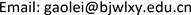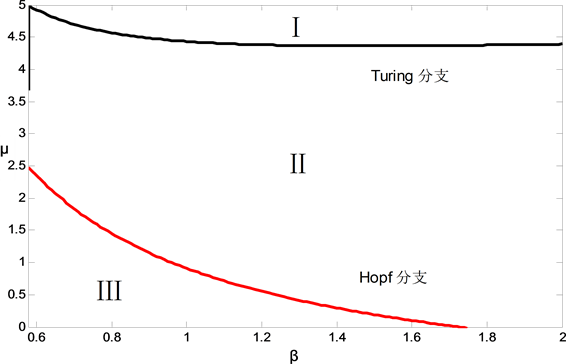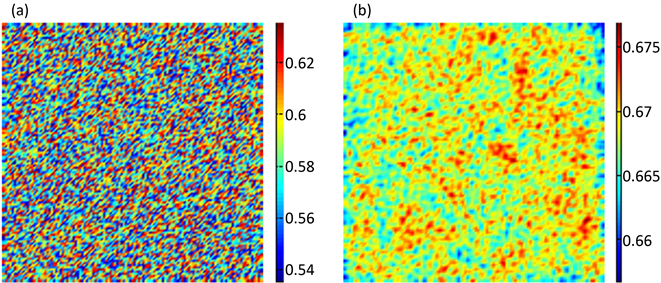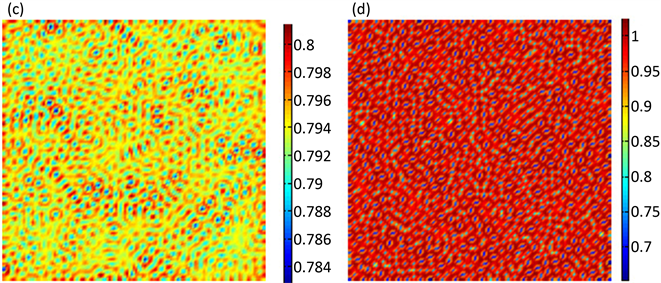﻿ 具有扩散项的互惠–寄生耦合模型图灵斑图研究 Analysis of Spatial Pattern in Mutualistic-Parasitic Coupled System with Diffusion

Vol.06 No.07(2017), Article ID:22432,9 pages
10.12677/AAM.2017.67101

Analysis of Spatial Pattern in Mutualistic-Parasitic Coupled System with Diffusion

Lei Gao

School of Mathematics and Information Science, Baoji University of Arts and Sciences, Baoji ShaanxiReceived: Oct. 3rd, 2017; accepted: Oct. 17th, 2017; published: Oct. 24th, 2017ABSTRACT

In this paper, we establish mutualistic-parasitic coupled spatial model incorporating diffusion, and study the dynamical behaviors of this spatial model. By spatial pattern theory, we obtain the conditions for Hopf bifurcation and Turing bifurcation, and give the exact parameter space for Turing domain. By utilizing difference approximation method, we find that the spatial model exhibits black eye spotted pattern, which shows that the diffusion can result in an isolated high density of parasite in the space.

Keywords:Mutualism, Parasitism, Bifurcation, Spatial Pattern, Diffusion1. 引言

2. 主要结果

2.1. 建立空间模型

$\left\{\begin{array}{l}\frac{\text{d}H}{\text{d}t}=H\left[{r}_{1}-{\alpha }_{1}H+\left(\mu -\frac{P}{H}\right)\beta P\right]\equiv F\left(H,P\right)\\ \frac{\text{d}P}{\text{d}t}=P\left({r}_{2}-{\alpha }_{2}\frac{P}{H}\right)\equiv G\left(H,P\right)\end{array}$ (1)

$\frac{\partial H}{\partial t}={d}_{1}{\nabla }^{2}H$$\frac{\partial P}{\partial t}={d}_{2}{\nabla }^{2}P$Table 1. Definitions and the symbols used in model (1)

$\left\{\begin{array}{l}\frac{\partial H}{\partial t}=F\left(H,P\right)+{d}_{1}{\nabla }^{2}H\\ \frac{\partial P}{\partial t}=G\left(H,P\right)+{d}_{2}{\nabla }^{2}P\end{array}$ (2)

2.2. 空间模型的分支分析

$\left\{\begin{array}{l}F\left({H}^{*},{P}^{*}\right)=0\\ G\left({H}^{*},{P}^{*}\right)=0\end{array}$ .

$\left\{\begin{array}{l}\frac{\partial h}{\partial t}={a}_{11}h+{a}_{12}p+{d}_{1}{\nabla }^{2}h\\ \frac{\partial p}{\partial t}={a}_{21}h+{a}_{22}p+{d}_{2}{\nabla }^{2}p\end{array}$ (3)

${a}_{11}={\frac{\partial F}{\partial H}|}_{\left({H}^{*},{P}^{*}\right)}=\frac{\left(\beta {\left({r}_{2}/{\alpha }_{2}\right)}^{2}-{\alpha }_{1}\right)\cdot {r}_{1}}{{\alpha }_{1}-\left(\mu -{r}_{2}/{\alpha }_{2}\right)\beta \cdot {r}_{2}/{\alpha }_{2}}$ ,

${a}_{12}={\frac{\partial F}{\partial P}|}_{\left({H}^{*},{P}^{*}\right)}=\frac{\left(\mu -\text{2}{r}_{2}/{\alpha }_{2}\right)\cdot \beta \cdot {r}_{1}}{{\alpha }_{1}-\left(\mu -{r}_{2}/{\alpha }_{2}\right)\beta \cdot {r}_{2}/{\alpha }_{2}}$ ,

${a}_{21}={\frac{\partial G}{\partial H}|}_{\left({H}^{*},{P}^{*}\right)}={r}_{2}^{2}/{\alpha }_{2}$ , ${a}_{22}={\frac{\partial G}{\partial P}|}_{\left({H}^{*},{P}^{*}\right)}=-{r}_{2}$ .

$\left(\begin{array}{l}h\\ p\end{array}\right)=\underset{\kappa }{\sum }\left(\begin{array}{l}{c}_{\kappa }^{1}\\ {c}_{\kappa }^{2}\end{array}\right){\text{e}}^{{\lambda }_{\kappa }t+i\kappa h}$ (4)

${\lambda }_{\kappa }\left(\begin{array}{l}{c}_{\kappa }^{1}\\ {c}_{\kappa }^{2}\end{array}\right)=\left(\begin{array}{cc}{a}_{11}-{\kappa }^{2}{d}_{1}& {a}_{12}\\ {a}_{21}& {a}_{22}-{\kappa }^{2}{d}_{2}\end{array}\right)\left(\begin{array}{l}{c}_{\kappa }^{1}\\ {c}_{\kappa }^{2}\end{array}\right)$ (5)

${\lambda }_{\kappa }^{2}-t{r}_{\kappa }{\lambda }_{\kappa }+{\Delta }_{\kappa }=0$

$t{r}_{\kappa }={a}_{11}+{a}_{22}-{\kappa }^{2}\left({d}_{1}+{d}_{2}\right)=t{r}_{0}-{\kappa }^{2}\left({d}_{1}+{d}_{2}\right)$

$\begin{array}{c}{\Delta }_{\kappa }={a}_{11}{a}_{22}-{a}_{21}{a}_{12}-{\kappa }^{2}\left({a}_{11}{d}_{2}+{a}_{22}{d}_{1}\right)+{\kappa }^{4}{d}_{1}{d}_{2}\\ ={\Delta }_{0}-{\kappa }^{2}\left({a}_{11}{d}_{2}+{a}_{22}{d}_{1}\right)+{\kappa }^{4}{d}_{1}{d}_{2}\end{array}$ ,

${\lambda }_{\kappa }=\frac{t{r}_{\kappa }±\sqrt{t{r}_{\kappa }^{2}-4{\Delta }_{\kappa }}}{2}$ .

1) 在跨临界分支中，非扩散模型的一个特征值消失。因此，解 ${\lambda }_{1}\cdot {\lambda }_{2}={\Delta }_{\kappa }=0$$\kappa =0$ ，可得跨临界分支参数 $\mu$ 的临界值表达式：

$\mu ={\alpha }_{1}{\alpha }_{2}/\left({r}_{2}\beta \right)+{r}_{2}/{\alpha }_{2}$ .

2) Hopf分支不稳定的出现对应于下面情况：对于非扩散模型来说，当根从负到正跨越实轴时产生了一对纯虚根。从数学角度看，当满足下列条件：

$Im\left({\lambda }_{\kappa }\right)\ne 0$ , $Re\left({\lambda }_{\kappa }\right)=0$ .

$\kappa =0$ 系统产生了Hopf分支，即

$t{r}_{0}={a}_{11}+{a}_{22}=0$ ,

$\left(\beta {\left({r}_{2}/{\alpha }_{2}\right)}^{2}-{\alpha }_{1}\right)\cdot {r}_{1}/\left({\alpha }_{1}-\left(\mu -{r}_{2}/{\alpha }_{2}\right)\beta \cdot {r}_{2}/{\alpha }_{2}\right)-{r}_{2}=0$ .

${r}_{1}\left(\beta {\left({r}_{2}/{\alpha }_{2}\right)}^{2}-{\alpha }_{1}\right)-{r}_{2}\left({\alpha }_{1}-\left(\mu -{r}_{2}/{\alpha }_{2}\right)\beta \cdot {r}_{2}/{\alpha }_{2}\right)=0$ ,

${r}_{1}\left(\beta {\left({r}_{2}/{\alpha }_{2}\right)}^{2}-{\alpha }_{1}\right)-{r}_{2}\left({\alpha }_{1}+\beta \cdot {\left({r}_{2}/{\alpha }_{2}\right)}^{2}\right)+\left({r}_{2}\cdot \beta \cdot {r}_{2}/{\alpha }_{2}\right)\mu =0$ ,

$\mu ={r}_{1}/{r}_{2}\cdot {\alpha }_{1}{\alpha }_{2}/\left({r}_{2}\beta \right)+{\alpha }_{1}{\alpha }_{2}/\left({r}_{2}\beta \right)+{r}_{2}/{\alpha }_{2}-{r}_{1}/{\alpha }_{2}$ .

3) 令

$B\left({\kappa }^{2}\right)=\left(\begin{array}{cc}{a}_{11}-{\kappa }^{2}{d}_{1}& {a}_{12}\\ {a}_{21}& {a}_{22}-{\kappa }^{2}{d}_{2}\end{array}\right)$ ,

$\mathrm{det}\left(B\left({\kappa }^{2}\right)\right)$ 是关于 ${\kappa }^{2}$ 的二次多项式，它在某些 ${\kappa }^{2}$ 值处取得极小值。首先假设非扩散模型在平衡点处是稳定的(特别的 $\mathrm{det}\left(B\left(0\right)\right)>0$ )，且图灵不稳定的发生需满足下面两个条件：(a) ${\kappa }^{2}>0$ (如果这个条件不成立，对于所有的 ${\kappa }^{2}=0$ ，由 $\mathrm{det}\left(B\left(0\right)\right)>0$ 可推出 $\mathrm{det}\left(B\left({\kappa }^{2}\right)\right)>0$ )是图灵不稳定的必要条件；(b) 图灵不稳定的充分条件是 $\mathrm{det}\left(B\left({\kappa }^{2}\right)\right)<0$ ，线性分析给出了图灵斑图产生的条件如下：

$t{r}_{0}={a}_{11}+{a}_{22}<0$ , ${\Delta }_{0}={a}_{11}{a}_{22}-{a}_{21}{a}_{12}>0$

${a}_{11}{d}_{2}+{a}_{22}{d}_{1}>0$${\left({a}_{11}{d}_{2}+{a}_{22}{d}_{1}\right)}^{2}>4{d}_{2}{d}_{1}\left({a}_{11}{a}_{22}-{a}_{21}{a}_{12}\right)$

$Im\left({\lambda }_{\kappa }\right)=0$ , $Re\left({\lambda }_{\kappa }\right)=0$ , $\kappa ={\kappa }_{T}\ne 0$ ,

${\kappa }_{T}^{2}=\sqrt{\frac{{a}_{11}{a}_{22}-{a}_{21}{a}_{12}}{{d}_{1}{d}_{2}}}$ .

${\lambda }_{{\kappa }_{T}}=\frac{t{r}_{{\kappa }_{T}}±\sqrt{t{r}_{{\kappa }_{T}}^{2}-4{\Delta }_{{\kappa }_{T}}}}{2}$

$\left\{\begin{array}{l}t{r}_{{\kappa }_{T}}=0\\ t{r}_{{\kappa }_{T}}^{2}-4{\Delta }_{{\kappa }_{T}}=0\end{array}$

$t{r}_{\kappa }={a}_{11}+{a}_{22}-{\kappa }_{T}^{2}\left({d}_{1}+{d}_{2}\right)$ ,

${\Delta }_{\kappa }={a}_{11}{a}_{22}-{a}_{21}{a}_{12}-{\kappa }_{T}^{2}\left({a}_{11}{d}_{2}+{a}_{22}{d}_{1}\right)+{\kappa }_{T}^{4}{d}_{1}{d}_{2}$ ,

$\left\{\begin{array}{l}{a}_{11}+{a}_{22}-{\kappa }_{T}^{2}\left({d}_{1}+{d}_{2}\right)=0\\ {a}_{11}{a}_{22}-{a}_{21}{a}_{12}-{\kappa }_{T}^{2}\left({a}_{11}{d}_{2}+{a}_{22}{d}_{1}\right)+{\kappa }_{T}^{4}{d}_{1}{d}_{2}=0\end{array}$ ,

$\left\{\begin{array}{l}{a}_{11}+{a}_{22}-{\kappa }_{T}^{2}\left({d}_{1}+{d}_{2}\right)=0\text{\hspace{0.17em}}\text{\hspace{0.17em}}\text{\hspace{0.17em}}\text{\hspace{0.17em}}\text{\hspace{0.17em}}\text{\hspace{0.17em}}\text{\hspace{0.17em}}\text{\hspace{0.17em}}\text{\hspace{0.17em}}\text{\hspace{0.17em}}\text{\hspace{0.17em}}\text{\hspace{0.17em}}\text{\hspace{0.17em}}\text{\hspace{0.17em}}\text{\hspace{0.17em}}\text{\hspace{0.17em}}\text{\hspace{0.17em}}\text{\hspace{0.17em}}\text{\hspace{0.17em}}\text{\hspace{0.17em}}\text{\hspace{0.17em}}\text{\hspace{0.17em}}\text{\hspace{0.17em}}\text{\hspace{0.17em}}\text{\hspace{0.17em}}\text{\hspace{0.17em}}\text{\hspace{0.17em}}\text{\hspace{0.17em}}\text{\hspace{0.17em}}\text{\hspace{0.17em}}\text{\hspace{0.17em}}\text{\hspace{0.17em}}\text{\hspace{0.17em}}\text{\hspace{0.17em}}\text{\hspace{0.17em}}\text{\hspace{0.17em}}\text{\hspace{0.17em}}\text{\hspace{0.17em}}\text{\hspace{0.17em}}\text{\hspace{0.17em}}\text{\hspace{0.17em}}\text{\hspace{0.17em}}\text{\hspace{0.17em}}\text{\hspace{0.17em}}\text{\hspace{0.17em}}\text{ }\text{ }\text{\hspace{0.17em}}\text{\hspace{0.17em}}\text{\hspace{0.17em}}\text{\hspace{0.17em}}\text{ }\text{ }\text{ }\text{\hspace{0.17em}}\text{\hspace{0.17em}}\text{\hspace{0.17em}}\text{\hspace{0.17em}}\text{\hspace{0.17em}}\text{\hspace{0.17em}}\text{\hspace{0.17em}}\text{\hspace{0.17em}}\text{\hspace{0.17em}}\text{\hspace{0.17em}}\text{\hspace{0.17em}}\text{\hspace{0.17em}}\text{\hspace{0.17em}}\left(6\right)\\ 2\left({a}_{11}{a}_{22}-{a}_{21}{a}_{12}\right)-{\kappa }_{T}^{2}\left({a}_{11}{d}_{2}+{a}_{22}{d}_{1}\right)=0\text{\hspace{0.17em}}\text{\hspace{0.17em}}\text{\hspace{0.17em}}\text{\hspace{0.17em}}\text{\hspace{0.17em}}\text{\hspace{0.17em}}\text{\hspace{0.17em}}\text{\hspace{0.17em}}\text{\hspace{0.17em}}\text{\hspace{0.17em}}\text{\hspace{0.17em}}\text{\hspace{0.17em}}\text{\hspace{0.17em}}\text{\hspace{0.17em}}\text{\hspace{0.17em}}\text{\hspace{0.17em}}\text{\hspace{0.17em}}\text{\hspace{0.17em}}\text{\hspace{0.17em}}\text{\hspace{0.17em}}\text{\hspace{0.17em}}\text{\hspace{0.17em}}\text{\hspace{0.17em}}\text{\hspace{0.17em}}\text{\hspace{0.17em}}\text{\hspace{0.17em}}\text{\hspace{0.17em}}\text{\hspace{0.17em}}\text{\hspace{0.17em}}\text{\hspace{0.17em}}\text{\hspace{0.17em}}\text{\hspace{0.17em}}\text{\hspace{0.17em}}\text{\hspace{0.17em}}\text{\hspace{0.17em}}\text{\hspace{0.17em}}\text{\hspace{0.17em}}\text{\hspace{0.17em}}\text{\hspace{0.17em}}\text{\hspace{0.17em}}\text{\hspace{0.17em}}\text{\hspace{0.17em}}\text{\hspace{0.17em}}\text{\hspace{0.17em}}\left(7\right)\end{array}$

$\left(7\right)×\left({d}_{1}+{d}_{2}\right)-\left(6\right)×\left({a}_{11}{d}_{2}+{a}_{22}{d}_{1}\right)$

$2\left({a}_{11}{a}_{22}-{a}_{21}{a}_{12}\right)\left({d}_{1}+{d}_{2}\right)-\left({a}_{11}+{a}_{22}\right)\left({a}_{11}{d}_{2}+{a}_{22}{d}_{1}\right)=0$ ,

$\left({a}_{11}-{a}_{22}\right)\left({a}_{22}{d}_{1}-{a}_{11}{d}_{2}\right)-2{a}_{12}{a}_{21}\left({d}_{1}+{d}_{2}\right)=0$ ,

$\begin{array}{l}\left(\frac{\left(\beta {\left({r}_{2}/{\alpha }_{2}\right)}^{2}-{\alpha }_{1}\right)\cdot {r}_{1}}{{\alpha }_{1}-\left(\mu -{r}_{2}/{\alpha }_{2}\right)\beta \cdot {r}_{2}/{\alpha }_{2}}+{r}_{2}\right)\left(-{r}_{2}\cdot {d}_{1}-\frac{\left(\beta {\left({r}_{2}/{\alpha }_{2}\right)}^{2}-{\alpha }_{1}\right)\cdot {r}_{1}}{{\alpha }_{1}-\left(\mu -{r}_{2}/{\alpha }_{2}\right)\beta \cdot {r}_{2}/{\alpha }_{2}}\cdot {d}_{2}\right)\\ -2\frac{\left(\mu -\text{2}{r}_{2}/{\alpha }_{2}\right)\cdot \beta \cdot {r}_{1}}{{\alpha }_{1}-\left(\mu -{r}_{2}/{\alpha }_{2}\right)\beta \cdot {r}_{2}/{\alpha }_{2}}\cdot {r}_{2}^{2}/{\alpha }_{2}\cdot \left({d}_{1}+{d}_{2}\right)=0\end{array}$ ,

$\begin{array}{l}\left(\beta {\left({r}_{2}/{\alpha }_{2}\right)}^{2}-{\alpha }_{1}\right)\cdot {r}_{2}{d}_{1}+{\left(\beta {\left({r}_{2}/{\alpha }_{2}\right)}^{2}-{\alpha }_{1}\right)}^{2}\cdot {r}_{1}/\left({\alpha }_{1}-\left(\mu -{r}_{2}/{\alpha }_{2}\right)\beta \cdot {r}_{2}/{\alpha }_{2}\right)\cdot {d}_{2}\\ +{r}_{2}^{2}\cdot {d}_{1}\left({\alpha }_{1}-\left(\mu -{r}_{2}/{\alpha }_{2}\right)\beta \cdot {r}_{2}/{\alpha }_{2}\right)/{r}_{1}+\left(\beta {\left({r}_{2}/{\alpha }_{2}\right)}^{2}-{\alpha }_{1}\right)\cdot {r}_{2}{d}_{2}\\ +2\beta \left(\mu -2{r}_{2}/{\alpha }_{2}\right)\cdot {r}_{2}^{2}/{\alpha }_{2}\left({d}_{1}+{d}_{2}\right)=0\end{array}$ ,

$\begin{array}{l}\left(\beta {\left({r}_{2}/{\alpha }_{2}\right)}^{2}-{\alpha }_{1}\right)\cdot \left({\alpha }_{1}-\left(\mu -{r}_{2}/{\alpha }_{2}\right)\beta \cdot {r}_{2}/{\alpha }_{2}\right)\cdot {d}_{1}/{d}_{2}\\ +{\left(\beta {\left({r}_{2}/{\alpha }_{2}\right)}^{2}-{\alpha }_{1}\right)}^{2}\cdot {r}_{1}/{r}_{2}+{r}_{2}/{r}_{1}\cdot {d}_{1}/{d}_{2}\cdot {\left({\alpha }_{1}-\left(\mu -{r}_{2}/{\alpha }_{2}\right)\beta \cdot {r}_{2}/{\alpha }_{2}\right)}^{2}\\ +\left(\beta {\left({r}_{2}/{\alpha }_{2}\right)}^{2}-{\alpha }_{1}\right)\cdot \left({\alpha }_{1}-\left(\mu -{r}_{2}/{\alpha }_{2}\right)\beta \cdot {r}_{2}/{\alpha }_{2}\right)\\ +2\beta \left(\mu -2{r}_{2}/{\alpha }_{2}\right)\cdot {r}_{2}/{\alpha }_{2}\cdot \left(1+{d}_{1}/{d}_{2}\right)\left({\alpha }_{1}-\left(\mu -{r}_{2}/{\alpha }_{2}\right)\beta \cdot {r}_{2}/{\alpha }_{2}\right)=0\end{array}$

$A=\beta \cdot {\left({r}_{2}/{\alpha }_{2}\right)}^{2}-{\alpha }_{1}$ , $D={d}_{1}/{d}_{2}$ , $r={r}_{1}/{r}_{2}$ ,

$\begin{array}{l}\left(1+D\right){\alpha }_{1}-\mu \beta {r}_{2}/{\alpha }_{2}\cdot \left(1+D\right)+\beta {\left({r}_{2}/{\alpha }_{2}\right)}^{2}\left(1+D\right)+Ar\\ +D/r\cdot \left({\alpha }_{1}^{2}-2{\alpha }_{1}\left(\mu -{r}_{2}/{\alpha }_{2}\right)\beta {r}_{2}/{\alpha }_{2}+{\left(\mu -{r}_{2}/{\alpha }_{2}\right)}^{2}{\beta }^{2}{\left({r}_{2}/{\alpha }_{2}\right)}^{2}\right)\\ +2\mu \beta {r}_{2}/{\alpha }_{2}\cdot \left(\left(1+D\right)/A\right)\left({\alpha }_{1}-\left(\mu -{r}_{2}/{\alpha }_{2}\right)\beta {r}_{2}/{\alpha }_{2}\right)\\ -4\beta {\left({r}_{2}/{\alpha }_{2}\right)}^{2}\left(\left(1+D\right)/A\right)\left({\alpha }_{1}-\left(\mu -{r}_{2}/{\alpha }_{2}\right)\beta {r}_{2}/{\alpha }_{2}\right)=0\end{array}$ .

$a={\beta }^{2}\cdot {\left({r}_{2}/{\alpha }_{2}\right)}^{2}\cdot \left(D/r-2\left(1+D\right)/A\right)$ ,

$b=-\beta \left({r}_{2}/{\alpha }_{2}\right)\cdot \left(1+D\right)+2{\alpha }_{1}\beta \cdot {r}_{2}/{\alpha }_{2}\cdot \left(\left(1+D\right)/A-D/r\right)+2{\beta }^{2}{\left({r}_{2}/{\alpha }_{2}\right)}^{3}\cdot \left(3\left(1+D\right)/A-D/r\right)$$c=\left(1+D\right)\cdot \left({\alpha }_{1}+\beta {\left({r}_{2}/{\alpha }_{2}\right)}^{2}\right)+Ar+D/r\cdot {\left(\left({\alpha }_{1}+\beta {\left({r}_{2}/{\alpha }_{2}\right)}^{2}\right)\right)}^{2}+4\beta {\left({r}_{2}/{\alpha }_{2}\right)}^{2}\cdot \left(1+D\right)$ .

$a{\mu }^{2}+b\mu +c=0$ ,

$\mu =\frac{-b±\sqrt{{b}^{2}-4ac}}{2a}$ .

3. 数值模拟

$\left\{\begin{array}{l}{H}^{k+1}\left(i,j\right)={H}^{k}\left(i,j\right)+\Delta t\cdot {F}_{1}\left(H,P\right)+\frac{\Delta t\cdot {d}_{1}}{{\left(\Delta h\right)}^{2}}\left[{H}^{k}\left(i+1,j\right)+{H}^{k}\left(i-1,j\right)+{H}^{k}\left(i,j+1\right)+{H}^{k}\left(i,j-1\right)-4{H}^{k}\left(i,j\right)\right]\\ {P}^{k+1}\left(i,j\right)={P}^{k}\left(i,j\right)+\Delta t\cdot {F}_{2}\left(H,P\right)+\frac{\Delta t\cdot {d}_{2}}{{\left(\Delta h\right)}^{2}}\left[{P}^{k}\left(i+1,j\right)+{P}^{k}\left(i-1,j\right)+{P}^{k}\left(i,j+1\right)+{P}^{k}\left(i,j-1\right)-4{P}^{k}\left(i,j\right)\right]\end{array}$Figure 1. The parameter space of bifurcationFigure 2. The time evolution of spatial pattern for parasitism

${F}_{1}\left(H,P\right)={H}^{k}\left(i,j\right)\cdot \left[{r}_{1}-{\alpha }_{1}{H}^{k}\left(i,j\right)+\left[\mu -\frac{{P}^{k}\left(i,j\right)}{{H}^{k}\left(i,j\right)}\right]\beta {P}^{k}\left(i,j\right)\right]$ . ${F}_{2}\left(H,P\right)={P}^{k}\left(i,j\right)\cdot \left[{r}_{2}-{\alpha }_{2}\frac{{P}^{k}\left(i,j\right)}{{H}^{k}\left(i,j\right)}\right]$

${H}_{n}\left({x}_{0},{y}_{j}\right)={H}_{n}\left({x}_{1},{y}_{j}\right),{H}_{n}\left({x}_{N+1},{y}_{j}\right)={H}_{n}\left({x}_{N},{y}_{j}\right)$ ,

${H}_{n}\left({x}_{i},{y}_{0}\right)={H}_{n}\left({x}_{i},{y}_{1}\right),{H}_{n}\left({x}_{i},{y}_{N+1}\right)={H}_{n}\left({x}_{i},{y}_{N}\right)$ ,

${P}_{n}\left({x}_{0},{y}_{j}\right)={P}_{n}\left({x}_{1},{y}_{j}\right),{P}_{n}\left({x}_{N+1},{y}_{j}\right)={P}_{n}\left({x}_{N},{y}_{j}\right)$ ,

${P}_{n}\left({x}_{i},{y}_{0}\right)={P}_{n}\left({x}_{i},{y}_{1}\right),{P}_{n}\left({x}_{i},{y}_{N+1}\right)={P}_{n}\left({x}_{i},{y}_{N}\right)$ .

Analysis of Spatial Pattern in Mutualistic-Parasitic Coupled System with Diffusion[J]. 应用数学进展, 2017, 06(07): 841-849. http://dx.doi.org/10.12677/AAM.2017.67101

1. 1. Anderson, R.M. and May, R.M. (1982) Coevolution of Hosts and Parasites. Parasitology, 85, 411-426. https://doi.org/10.1017/S0031182000055360

2. 2. Best, A., White, A., Kisdi, É., Antonovics, J., Brockhurst, M.A. and Boots, M. (2010) The Evolution of Host-Parasite Range. American Naturalist, 176, 63-71. https://doi.org/10.1086/653002

3. 3. Roepstorff, A., Mejer, H., Nejsum, P. and Thamsborg, S.M. (2011) Helminth Parasites in Pigs: New Challenges in Pig Production and Current Research Highlights. Veterinary Parasitology, 180, 72-81. https://doi.org/10.1016/j.vetpar.2011.05.029

4. 4. Ågren, J.A. and Wright, S.I. (2011) Co-Evolution between Transposable Elements and Their Hosts: A Major Factor in Genome Size Evolution? Chromosome Research, 19, 776-786. https://doi.org/10.1007/s10577-011-9229-0

5. 5. Ravensdale, M., Nemri, A., Thrall, P.H., Ellis, J.G. and Dodds, P.N. (2011) Co-Evolutionary Interactions between Host Resistance and Pathogen Effector Genes in Flax Rust Disease. Molecular Plant Pathology, 12, 93-102. https://doi.org/10.1111/j.1364-3703.2010.00657.x

6. 6. Britton, J.R., Pegg, J. and Williams, C.F. (2011) Pathological and Eco-logical Host Consequences of Infection by an Introduced Fish Parasite. PloS One, 6, e26365. https://doi.org/10.1371/journal.pone.0026365

7. 7. Sun, G.Q., Jusup, M., Jin, Z., Wang, Y., and Wang, Z. (2016) Pattern Tran-sitions in Spatial Epidemics: Mechanisms and Emergent Properties. Physics of Life Reviews, 19, 43-73.

8. 8. 徐弘君, 程少博. 肠寿: 肠道健康新生活[M]. 北京: 清华大学出版社, 2005: 19-35.

9. 9. 王俊丽, 聂国兴. 低聚木糖与肠道微生态研究进展[J]. 世界华人消化杂志, 2011, 19(7): 710-717.

10. 10. 高磊, 杨燕, 贺军州, 等. 互惠–寄生耦合系统的稳定性[J]. 生态学报, 2012, 32(21):6848-6855.

11. 11. 欧阳颀. 反应扩散系统中的斑图动力学[M]. 上海: 上海科技教育出版社, 2000: 10-70.

12. 12. 孙桂全. 传染病模型的时空复杂性研究[D]: [硕士学位论文]. 太原: 中北大学, 2009.